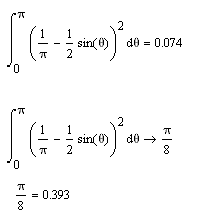## Symbolic Integration bug in Mathcad 14

April 4th, 2008 | Categories: mathcad, mathematica | Tags:

Just a quick one – someone sent me the following Mathcad bug report and so I thought I would share it. No problems with it in Mathematica or Matlab (using the symbolic toolbox). I find symbolic integration bugs interesting – especially if they come from one of the well known commercial packages such as Mathematica, Maple, Matlab or Mathcad. If you ever come across any then please do let me know in the comments.

The correct answer is Pi/8- 1/Pi which evaluates to about 0.074 so you can see from the image below that Mathcad gets the correct numerical result but fails to get the symbolic result.1. Use Mathcad 14 Build M011. In the menu, use symbolics\simplify to export the result without integrals and then simplify it again to get the required result.

2. Hi,

Okay here’s what may be a similar bug, but in Matlab:

syms x c w
int(1/(c*sin(w*x)+c+1)^2)

Now plot the result:

x = 0:.01:2*pi;
figure, c = 100; w = 1;

% Integral:
y1 = 8./w./(c+1).*c.^2./(8.*c+4)./(2.*c.*tan(1./2.*w.*x)+c.*tan(1./2.*w.*x).^2+c+tan(1./2.*w.*x).^2+1).*tan(1./2.*w.*x)+ …
8./w./(c+1).*c./(8.*c+4)./(2.*c.*tan(1./2.*w.*x)+c.*tan(1./2.*w.*x).^2+c+tan(1./2.*w.*x).^2+1)+ …
8./w./(c+1).*c.^2./(8.*c+4)./(2.*c.*tan(1./2.*w.*x)+c.*tan(1./2.*w.*x).^2+c+tan(1./2.*w.*x).^2+1)+ …
8./w./(c+1).*c.^2./(8.*c+4)./(2.*c+1).^(1./2).*atan(1./2.*(2.*(c+1).*tan(1./2.*w.*x)+2.*c)./(2.*c+1).^(1./2))+ …
2./w./(c+1)./(2.*c+1).^(1./2).*atan(1./2.*(2.*(c+1).*tan(1./2.*w.*x)+2.*c)./(2.*c+1).^(1./2));

% Or, simplified:
y2 = (c*tan(1/2*w*x) + 1 + c).*(8/w/(c+1)*c/(8*c+4)./(2*c*tan(1/2*w*x)+c*tan(1/2*w*x).^2+c+tan(1/2*w*x).^2+1)) + …
(4*c^2/(8*c+4)+1)*2/w/(c+1)./(2*c+1)^(1/2).*atan(1/2*(2*(c+1)*tan(1/2*w*x)+2*c)/(2*c+1)^(1/2));

subplot (3,1,1), plot (x,1./(c*sin(w*x)+c+1).^2,’.’)
subplot (3,1,2), plot (x,y1,’.’)
subplot (3,1,3), plot (x,y2,’.’)

3. I have since found a workaround. Refer to, for example, the works of D.J. Jeffrey at the University of Western Ontario, Canada. A good starting place is “Rectifying transformations for the integration of rational trigonometric functions”, J Symbolic Computation 24: 563-73, 1997. His 1993 and 1994 papers are also useful.

One day they might even be incorporated into symbolic integration software so we don’t need to do this ourselves!

4. Ifound a bug in mathcad13.
I have 2-dinensional vectors represented as spinors: 1) vector a with real coordinates 2)vector k(x) which has upper coordinate 1 and down equal to x. I multiply them. Then I find that the products
a*k(2i) and k(2i)*a are complex conjugate. The square of a vector is always real, as if one of them is taken complex conjugate. I don’t need hermitian conjugation, but I cannot get rid of it.

5. Use Maxima, it produces the correct symbolic and numerical answers in a Windows (and other platform) GUI.

6. Maxima code, enter these three expressions:

(1/%pi-1/2*sin(theta))^2;integrate(%, theta, 0, %pi);

float(%), numer;

wxplot2d(%i1, [theta,0,%pi]);

Note: % means previous expression and %pi means PI